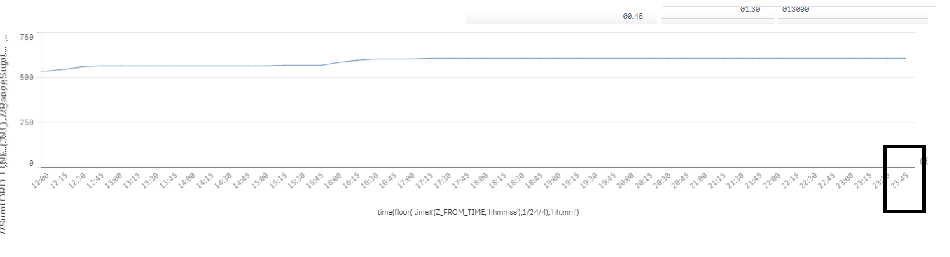# New to Qlik Sense

Discussion board where members can get started with Qlik Sense.

Announcements
Qlik® Product Spotlight: Discover what’s possible. Get more from our products.
See for yourself. Register today.
Contributor II

## If Clause is creating Issue in Rangesum

Hi All,

I need to show rangesum for maxdate in dashboard against Time in X axis in 15 mins interval. And my expression is below:

RangeSum(Above(Sum({\$<Z_DATE=, M_DATE={'\$(=(Date('\$(vDATE)','M/D/YYYY')))'}>} ORD_LINE_CNT),0,RowNo()))vDATE is for max date. For max date we have data till 17:15. Chart is showing data till 17:15. as expected.

But if we mention below expression then chart is showing data for all time , i.e. till 23:45.

If (Selector = 'Cum',RangeSum(Above(Sum({\$<Z_DATE=, M_DATE={'\$(=(Date('\$(vDATE)','M/D/YYYY')))'}>} ORD_LINE_CNT),0,RowNo())),0)Thanks in advance for help.

Regards,
Sarif

1 Solution

Accepted SolutionsMVP

## Re: If Clause is creating Issue in Rangesum

The idea is that because we added this set analysis {\$<Z_DATE=, M_DATE={'\$(=(Date('\$(vDATE)','M/D/YYYY')))'}>} the Avg expression one equal to 1 for when the hours meet the criteria in your set analysis and when they don't Avg expression = 0. So I am multiplying with either 1 or 0. Does this make sense?

Let me know if you are still confusedIf you are clear, then I would request you to close this thread by marking correct and helpful responses.

Best,

Sunny

9 RepliesMVP

## Re: If Clause is creating Issue in Rangesum

If(Only({\$<Z_DATE=, M_DATE={'\$(=(Date('\$(vDATE)','M/D/YYYY')))'}>} Selector) = 'Cum',

RangeSum(Above(Sum({\$<Z_DATE=, M_DATE={'\$(=(Date('\$(vDATE)','M/D/YYYY')))'}>} ORD_LINE_CNT),0,RowNo())))

Contributor II

## Re: If Clause is creating Issue in Rangesum

Hello Sunny,

It is also showing all time....not restricting till 17:15.

Thanks,

SarifMVP

## Re: If Clause is creating Issue in Rangesum

If(Selector = 'Cum',

RangeSum(Above(Sum({\$<Z_DATE=, M_DATE={'\$(=(Date('\$(vDATE)','M/D/YYYY')))'}>} ORD_LINE_CNT),0,RowNo()))) * Avg({\$<Z_DATE=, M_DATE={'\$(=(Date('\$(vDATE)','M/D/YYYY')))'}>} 1)

Contributor II

## Re: If Clause is creating Issue in Rangesum

Thanks Sunny..it is working fine...one more request. I trying to incorporate else clause as well. Like if Selector = 'Cum', then rangesum else it will be normal sum. Like:

If(Selector = 'Cum',

RangeSum(Above(Sum({\$<Z_DATE=, M_DATE={'\$(=(Date('\$(vDATE)','M/D/YYYY')))'}>} ORD_LINE_CNT),0,RowNo())), Sum({\$<Z_DATE=, M_DATE={'\$(=(Date('\$(vDATE)','M/D/YYYY')))'}}>} ORD_LINE_CNT) )*

Avg({\$<Z_DATE=, M_DATE={'\$(=(Date('\$(vDATE)','M/D/YYYY')))'}>}1)

This is not working properly. Could you please help me out on this.

Thanks a lot for all your help.

Contributor II

## Re: If Clause is creating Issue in Rangesum

Hey Sunny..now it is working fine. My expression was wrong. Sorry for that. Thanks a lot. But could you please explain in short how it is working when we are multiplying with AVG function.

I checked that the AVG function is returning 1. And I tired to multiply the same expression with 1 instead of AVG function, the it was not working properly. It was showing all the available time.

It would be really greatfull if you find some time and let me know how this is working.

Thanks again.

SarifMVP

## Re: If Clause is creating Issue in Rangesum

The idea is that because we added this set analysis {\$<Z_DATE=, M_DATE={'\$(=(Date('\$(vDATE)','M/D/YYYY')))'}>} the Avg expression one equal to 1 for when the hours meet the criteria in your set analysis and when they don't Avg expression = 0. So I am multiplying with either 1 or 0. Does this make sense?

Let me know if you are still confusedIf you are clear, then I would request you to close this thread by marking correct and helpful responses.

Best,

Sunny

Contributor II

## Re: If Clause is creating Issue in Rangesum

Dear Sunny,

I am bit confused..it would be really helpful for me if you explain this in bit details...

Thanks,

Sarif

Contributor II

## Re: If Clause is creating Issue in Rangesum

Hey Suuny.. I got the idea..thanks a bro..MVP

## Re: If Clause is creating Issue in Rangesum

No problem, I apologize for not able to go over in further details after my last post. I was planning to do that soon, but if you already got it, then I might notCommunity Browser